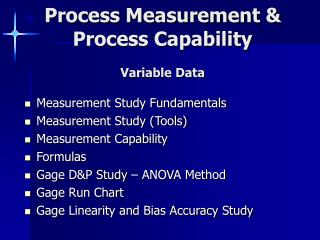# Process Measurement & Process Capability Variable Data - PowerPoint PPT PresentationDownload PresentationProcess Measurement & Process Capability Variable Data

Process Measurement & Process Capability Variable DataDownload Presentation## Process Measurement & Process Capability Variable Data

- - - - - - - - - - - - - - - - - - - - - - - - - - - E N D - - - - - - - - - - - - - - - - - - - - - - - - - - -
##### Presentation Transcript

1. Process Measurement & Process CapabilityVariable Data • Measurement Study Fundamentals • Measurement Study (Tools) • Measurement Capability • Formulas • Gage D&P Study – ANOVA Method • Gage Run Chart • Gage Linearity and Bias Accuracy Study

2. Measurement Study Fundamentals • Measurement System can have both • Bias • Variation • Formulas • Bias: µ observed = µactual (part) + µmeasurement system • Variation:  2 observed (total) =  2 o actual (part) +  2measurement system Where µis the mean  2 is the variance

3. Possible Sources of Variation for a Process and Its Measurement System Parts (Example) • Observations • Measurements • Data

4. Definition of Terms • Linearity • A measure of how the size of a part affects the accuracy of the measurement system. It is the difference in the observed accuracy values through the expected range of measurements. • Accuracy • A measure of the bias in the measurement system. It is the difference between the observed measurement and a master value. • Stability (Drift) • A measure of how accurately a system performs over time. It is the total variation obtained with a particular measurement instrument, on the same part, when measuring a single characteristic over time. • Precision • Precision or measurement variation is the variation due to a measurement system and can be broken down into the following two components: • Repeatability • The variation observed when the same operatior measures the sam part repeatedly with the same device. • Reproductibility • The variation observed when different operators measure the same parts using the same insturment.

5. Measurement Issues • The bottom line is that we really want to answer the following questions: • How large is the measurement bias? • How big is the measurement variation? • What are the sources of bias and measurement variation? • Is the measurement instrument stable over time? • How capable is the instrument for measuring the part alignment? • How can we improve the measurement system?

6. Measurement Study (Tools) • Gage Run chart: • A plot of all observations by operator and part number. A horizontal reference line is drawn at the mean, which can be calculated from the data, or a value can be entered from prior knowledge of the process. You can use this chart to quickly assess differences in measurements between different operators and parts. A stable process would give you a random horizontal scattering of pointsw; an operator or part effect would give you some kind of pattern in the plot. • Gage R&R Study: • Determines how much of the obgserved process variation is due to measurement system variation. Either of two methods can be used to perform this analysis, the latter being more informative: (a) X and R, or (b) ANOVA (Analysis of Variance). The X and R method breaks down the overall variation into three categories: (1)part-to-part, (2) repeatability, and (3) reproducibility. The ANOVA method goes one step further and breaks down reproducibility into its operator and operator by part interaction. • Gage Lineaity and Accuracy Study: • Tells how accurate the measurements are through the expected range of the measurements. It answers the question, "Does the gage have the same accuracy for all sizes of parts or objects being measured?" A gage accuracy study examines the difference between the observed average measurement and a reference or master value. It answers the question, "How accurate is the measurement instrument when compared to a master value?"

7. Measurement Capability • Precision-to-Tolerance (P/T) Ratio: • It is usually 5.15 * total gage R&R standard deviation (obtained from the ouptut of the Gage R&R Study) divided by the tolerance, I.e., Spring 2006 Page 5 of 14 Process Measurement – Classroom Exercise where USL, LSL are the upper and lower specification limits respectively If the P/T ratio is <10%, the measurement instrument is considered acceptable; if it is between 10% and 30%, it is marginal; and greater than 30% it is unacceptable."

8. FormulasGage R&R Study ANOVA Method

9. F Tests

10. Gage R&R Table . . . Var Comp for ANOVA Method

11. (Operator * Part Not included)

12. Number of Distinct Categories (Resolution) • Represents number of non-overlapping confidence intervals that will span the range of product variation. Think of it as the number of groups within process data that measurement system can discern: Rule: Good number should be equal to or greater than 5.

13. Gage Run Chart • Shows • Part-to-Part Variation • Operator-to-Operator Variation • Repeatability (Variation within each data group)

14. Gage Linearity & Bias (Accuracy) Study • Linearity • Measures how accurate measurements are through their expected range. It answers the question, "Does my gage have the same accuracy for all sizes of objects being measured?" • % Linearity • Linearity expressed as a percent of the overall process variation

15. Gage Bias • Bias • The actual measurment minus the corresponding reference value • % Bias • Bias expressed as a percent of the overall process variation

16. End